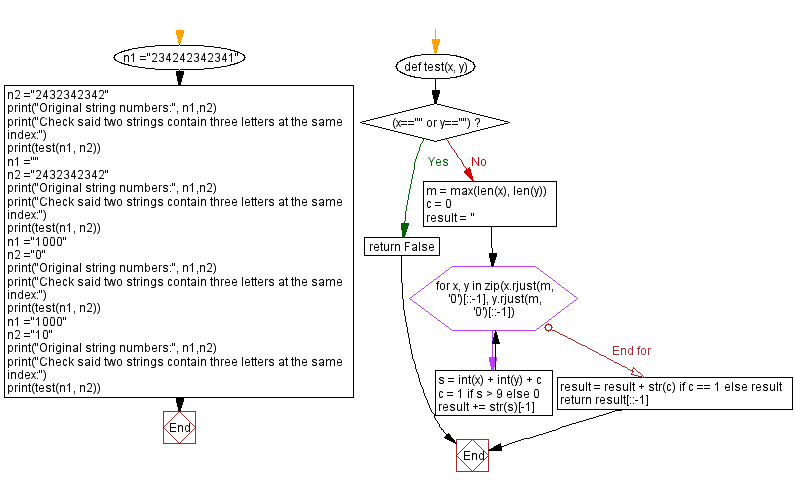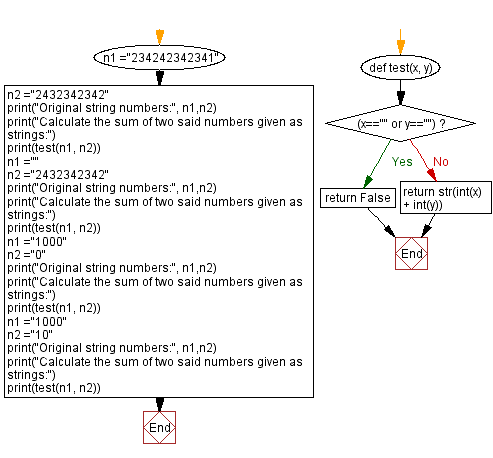﻿ Python - Calculate the sum of two numbers given as strings# Python Exercises: Calculate the sum of two numbers given as strings

## Python String: Exercise-112 with Solution

Write a Python program to calculate the sum of two numbers given as strings. Return the result in the same string representation.

Sample Data:
( “234242342341”, “2432342342”) -> “236674684683”
( “”, “2432342342”) -> False ( “1000”, “0”) -> “1000”
( “1000”, “10”) -> “1010”

Sample Solution-1:

Python Code:

``````def test(x, y):
if (x=="" or y==""):
return False
m = max(len(x), len(y))
c = 0
result = ''
for x, y in zip(x.rjust(m, '0')[::-1], y.rjust(m, '0')[::-1]):
s = int(x) + int(y) + c
c = 1 if s > 9 else 0
result += str(s)[-1]

result = result + str(c) if c == 1 else result
return result[::-1]

n1 ="234242342341"
n2 ="2432342342"
print("Original string numbers:", n1,n2)
print("Check said two strings contain three letters at the same index:")
print(test(n1, n2))
n1 =""
n2 ="2432342342"
print("Original string numbers:", n1,n2)
print("Check said two strings contain three letters at the same index:")
print(test(n1, n2))
n1 ="1000"
n2 ="0"
print("Original string numbers:", n1,n2)
print("Check said two strings contain three letters at the same index:")
print(test(n1, n2))
n1 ="1000"
n2 ="10"
print("Original string numbers:", n1,n2)
print("Check said two strings contain three letters at the same index:")
print(test(n1, n2))
```
```

Sample Output:

```Original string numbers: 234242342341 2432342342
Check said two strings contain three letters at the same index:
236674684683
Original string numbers:  2432342342
Check said two strings contain three letters at the same index:
False
Original string numbers: 1000 0
Check said two strings contain three letters at the same index:
1000
Original string numbers: 1000 10
Check said two strings contain three letters at the same index:
1010
```

Flowchart:## Visualize Python code execution:

The following tool visualize what the computer is doing step-by-step as it executes the said program:

Sample Solution-2:

Python Code:

``````def test(x, y):
if (x=="" or y==""):
return False
return str(int(x) + int(y))

n1 ="234242342341"
n2 ="2432342342"
print("Original string numbers:", n1,n2)
print("Calculate the sum of two said numbers given as strings:")
print(test(n1, n2))
n1 =""
n2 ="2432342342"
print("Original string numbers:", n1,n2)
print("Calculate the sum of two said numbers given as strings:")
print(test(n1, n2))
n1 ="1000"
n2 ="0"
print("Original string numbers:", n1,n2)
print("Calculate the sum of two said numbers given as strings:")
print(test(n1, n2))
n1 ="1000"
n2 ="10"
print("Original string numbers:", n1,n2)
print("Calculate the sum of two said numbers given as strings:")
print(test(n1, n2))
```
```

Sample Output:

```Original string numbers: 234242342341 2432342342
Calculate the sum of two said numbers given as strings:
236674684683
Original string numbers:  2432342342
Calculate the sum of two said numbers given as strings:
False
Original string numbers: 1000 0
Calculate the sum of two said numbers given as strings:
1000
Original string numbers: 1000 10
Calculate the sum of two said numbers given as strings:
1010
```

Flowchart:## Visualize Python code execution:

The following tool visualize what the computer is doing step-by-step as it executes the said program:

Python Code Editor:

Have another way to solve this solution? Contribute your code (and comments) through Disqus.

Previous Python Exercise: Alphabet position in a string.
Next Python Exercise: Sort a string based on its first character.

What is the difficulty level of this exercise?

Test your Programming skills with w3resource's quiz.

﻿

## Python: Tips of the Day

Getting sorted iterable (can sort by "compare" function):

```>>> a = [1, 2, -3]
>>> sorted(a)
[-3, 1, 2]

>>> sorted(a,key=abs)
[1, 2, -3]
```